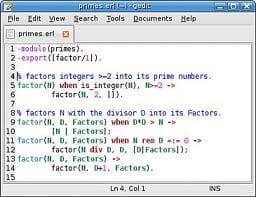0
Thanks

A few words of thanks would be greatly appreciated.

# Calculate the exponential by recursion

## Calculate the exponential by recursion## Issue

Please, I would like to know the error (s) that exists in this algorithm. For information: this algorithm is to compute the exponential method by recursion.

```#include <stdio.h>
#include <stdlib.h>
#include <math.h>

int factoriel(int n, int i, int fact)
{
if(i=n+1)
{
fact=1;
return fact;
}
else
{
fact = fact*i;
i++;
factoriel(n,i,fact);
}
}

float puissance(int n, int i, float x, float p)
{
if(i=n+1)
{
p=1;
return p;
}
else
{
p=p*x;
i++;
puissance(n,i,x,p);
}
}

int main()
{
const float EPS=0.0001;
int i, n, fact;
float x,p,s,T;

printf("Donner x: ");
scanf("%f", &x);
n=0; T=1; s=0; fact=1; p=1;
while(floor(T) > EPS)
{
T=puissance(n,1,x,p)/factoriel(n, 1, fact);
s=s+T;
n=n+1;
}
printf("Exp(%f) = %f\n", x, s);
}```

## Solution

Several errors.

I pass over the absurdity of using recursion to calculate the factorial and power, I suppose you requested.

First, detailed syntax ultra-classic: the test is ==, not =. In your two functions should be if (i == n +1)

Worse, in the else test both functions, there is no return. So your function returns nothing, it may take a random value. I guess the idea was to have the first return factorial (n, i, fact);
But even with this correction factor and Thy power will not work. You make fine calculations, then returned to the stage n +1, you can force the result to 1!
Finally, in your hand, you have reinitialize p and fact on each loop.

In my opinion it is better to write the factorial function and power to work by decreasing values (from n to 1) rather than increasing from 1 to n +1. Function only requires one parameter, it is much simpler (no i, Fact, or p)

## Note

Solve by le père
0
Thanks

A few words of thanks would be greatly appreciated.